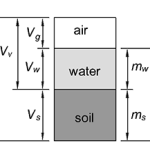## How to Calculate Soil PorosityThe soil porosity, also called the void fraction, is the ratio of the volume of voids (open spaces, i.e. air and water) in a soil to the total volume. Where: Vv = Volume of voids (m3 or ft3) Vt = Total Volume (m3 or ft3) Vg = Volume of air (m3 or ft3) Vw = […]